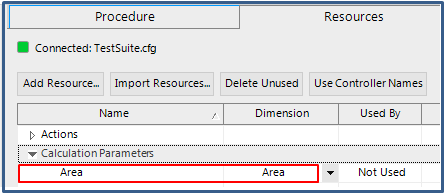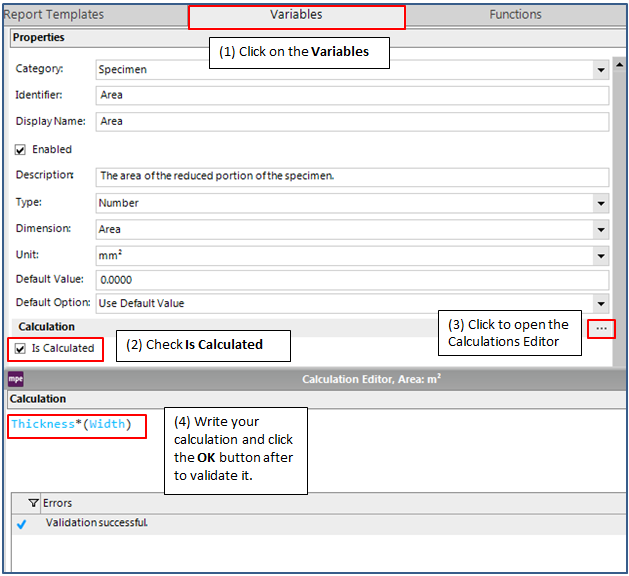# How can I change a calculation parameter(s) for a imported calculated float signal in TestSuite without having to do this in the Calculations Editor window in 793 Station Manager?

QUESTION

How can I change a calculation parameter(s) for a imported calculated float signal in TestSuite without having to do this in the Calculations Editor window in 793 Station Manager?

The calculation parameter Area will be used in this tutorial but the concept is the same for any calculation parameter that may change in each test run.

1. Go to the Resources tab in TestSuite and click on Import Resources and select Import the selected controller resources… (Figure 1).Figure 1 – Import Resources in TestSuite

1. Expand Calculation Parameters and choose the calculation parameter Area (Figure 2).Figure 2 – Selecting a calculation parameter to import to TestSuite

1. Click on OK so it becomes available under your Calculation Parameters in your resource list TestSuite (Figure 3).Figure 3 – Calculation parameter successfully imported to TestSuite

1. Make the variable Area in TestSuite a calculated variable; Area=Width *Thickness (Figure 4).Figure 4 – Creating a calculated variable

1. Add an Input Variables activity near the beginning of your test procedure so that the operator is prompted to input values for Width (W) and Thickness (B).  (Figure 5).Figure 5 – Adding an Input Variables activity in the test procedure

6.  Add a Calculate Variables: activity after the Input Variables: activity to calculate the variable Area (Figure 6).Figure 6 - Calculate Variables activity

7.  Add a Set Calculation Parameter: activity after your Input Variables: activity.  Assign the imported resource Calculation Parameter Area to equal the calculated variable called Area in TestSuite (Figure 7).Figure 7 – Assigning the value of the calculated variable Area to the Calculation parameter Area

K
Keary is the author of this solution article.

Did you find it helpful? Yes No

Send feedback